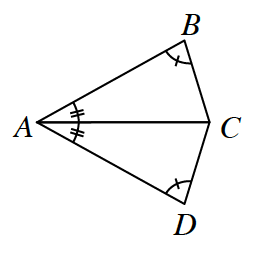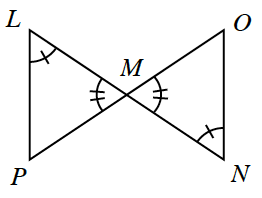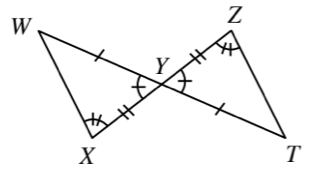### Home > INT1 > Chapter 7 > Lesson 7.1.4 > Problem7-46

7-46.

For each pair of triangles below, determine if the triangles are congruent. If the triangles are congruent,

• Complete the congruence statement

• State the congruence property

• Determine a sequence of rigid transformations that maps one triangle onto the other

Otherwise, explain why you cannot conclude that the triangles are congruent. Note that the figures are not necessarily drawn to scale.

1. $ΔABC ≅ Δ\text{ _____}$$ΔADC$; $\text{AAS} ≅$ (Remember that $AC=CA$ by the  reflexive property.)

1. $ΔPLM ≅ Δ\text{ _____}$No solution; only the angles are congruent. These triangles can only be proved to be similar by $\text{AA}∼$ but not congruent.

1. $ΔWXY ≅ Δ\text{ _____}$$ΔTZY$; $\text{SAS} ≅$ or $\text{AAS}≅$.